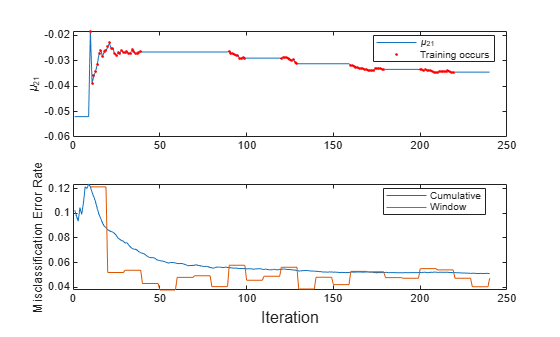Perform Conditional Training During Incremental Learning

This example shows how to train a naive Bayes multiclass classification model for incremental learning only when the model performance is unsatisfactory.

The flexible incremental learning workflow enables you to train an incremental model on an incoming batch of data only when it is necessary (see What Is Incremental Learning?). For example, if the performance metrics of a model are satisfactory, then, to increase efficiency, you can skip training on incoming batches until the metrics become unsatisfactory.

Load the human activity data set. Randomly shuffle the data.

n = numel(actid);
rng(1) % For reproducibility
idx = randsample(n,n);
X = feat(idx,:);
Y = actid(idx);

For details on the data set, enter Description at the command line.

Train Naive Bayes Classification Model

Configure a naive Bayes classification model for incremental learning by setting all the following:

• The maximum number of expected classes to 5

• The tracked performance metric to the misclassification error rate, which also includes minimal cost

• The metrics window size to 1000

• The metrics warmup period to 50

initobs = 50;
Mdl = incrementalClassificationNaiveBayes('MaxNumClasses',5,'MetricsWindowSize',1000,...
'Metrics','classiferror','MetricsWarmupPeriod',initobs);

Fit the configured model to the first 50 observations.

Mdl = fit(Mdl,X(1:initobs,:),Y(1:initobs))
Mdl =
incrementalClassificationNaiveBayes

IsWarm: 1
Metrics: [2x2 table]
ClassNames: [1 2 3 4 5]
ScoreTransform: 'none'
DistributionNames: {1x60 cell}
DistributionParameters: {5x60 cell}

Properties, Methods

haveTrainedAllClasses = numel(unique(Y(1:initobs))) == 5
haveTrainedAllClasses = logical
1

Mdl is an incrementalClassificationNaiveBayes model object. The model is warm (IsWarm is 1) because all the following conditions apply:

• The initial training data contains all expected classes (haveTrainedAllClasses is true).

• Mdl was fit to Mdl.MetricsWarmupPeriod observations.

Therefore, the model is prepared to generate predictions, and incremental learning functions measure performance metrics within the model.

Perform Incremental Learning with Conditional Training

Suppose that you want to train the model only when the most recent 1000 observations have a misclassification error greater than 5%.

Perform incremental learning, with conditional training, by following this procedure for each iteration:

1. Simulate a data stream by processing a chunk of 100 observations at a time.

2. Update the model performance by passing the model and current chunk of data to updateMetrics. Overwrite the input model with the output model.

3. Fit the model to the chunk of data only when the misclassification error rate is greater than 0.05. Overwrite the input model with the output model when training occurs.

4. Store the misclassification error rate and the mean of the first predictor in the second class ${\mu }_{21}$ to see how they evolve during training.

5. Track when fit trains the model.

% Preallocation
numObsPerChunk = 100;
nchunk = floor((n - initobs)/numObsPerChunk);
mu21 = zeros(nchunk,1);
ce = array2table(nan(nchunk,2),'VariableNames',["Cumulative" "Window"]);
trained = false(nchunk,1);

% Incremental fitting
for j = 1:nchunk
ibegin = min(n,numObsPerChunk*(j-1) + 1 + initobs);
iend   = min(n,numObsPerChunk*j + initobs);
idx = ibegin:iend;
Mdl = updateMetrics(Mdl,X(idx,:),Y(idx));
ce{j,:} = Mdl.Metrics{"ClassificationError",:};
if ce{j,"Window"} > 0.05
Mdl = fit(Mdl,X(idx,:),Y(idx));
trained(j) = true;
end
mu21(j) = Mdl.DistributionParameters{2,1}(1);
end

Mdl is an incrementalClassificationNaiveBayes model object trained on all the data in the stream.

To see how the model performance and ${\mu }_{21}$ evolved during training, plot them on separate subplots. Identify periods during which the model was trained.

subplot(2,1,1)
plot(mu21)
hold on
plot(find(trained),mu21(trained),'r.')
ylabel('\mu_{21}')
legend('\mu_{21}','Training occurs','Location','best')
hold off
subplot(2,1,2)
plot(ce.Variables)
ylabel('Misclassification Error Rate')
xlabel('Iteration')
legend(ce.Properties.VariableNames,'Location','best')The trace plot of ${\mu }_{21}$ shows periods of constant values, during which the model performance within the previous 1000 observation window is at most 0.05.